# KCET 2016 Mathematics Paper with Solutions

Karnataka Common Entrance Test is popularly known as KCET. It is an examination conducted every year for admission into various institutions in the state of Karnataka. The KCET 2016 Mathematics paper solved by the experts at BYJU’S is included on this page. The KCET aspirants are advised to practise these questions to understand the coverage of topics as well as the overall exam pattern for the upcoming exam. Solving sample question papers also helps the candidates improve their problem -solving speed.

### KCET 2016 - Mathematics

1. The Set A has 4 elements and the set B has 5 elements then the number of injective mappings that can be defined from A to B is

1. a. 144
2. b. 72
3. c. 60
4. d. 120

Solution:

No. of elements in set A = 4

No. of elements in set B = 5

No. of injective mapping = 5P4 = (5!/1!) = 120

2. Let f: R -> R be defined by f(x) =2x+6 which is a bijective mapping then f–1(x) is given by A

1. a. (x/2) -3
2. b. 2x+6
3. c. x–3
4. d. 6x+2

Solution:

f(x) = 2x+ 6

y = 2x+ 6

y – 6 = 2x

$$\frac{y-6}{2}=x\left \{ put \, y=x \, and \, x = f^{-1}(x) \right \}$$

$$f^{-1}(x)=\frac{x}{2}-3$$

3. Let * be binary operation defined on R by

$$a*b=\frac{a+b}{4}\forall a,b\epsilon R$$
then the operation is *

1. a. Commutative and Associative
2. b. Commutative but not Associative
3. c. Associative but not Commutative
4. d. Neither Associative nor Commutative

Solution:

$$a*b =\frac{a+b}{2}(given)$$

$$\frac{a+b}{4}=\frac{b+a}{4}$$

The given operation is commutative

Associative property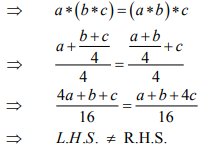The given operation is not associative.

4. The value of

$$sin^{-1}\left ( cos\frac{53\pi }{5} \right )$$

1. a.
$$\frac{3\pi }{5}$$
2. b.
$$\frac{-3\pi }{5}$$
3. c.
$$\frac{\pi }{10}$$
4. d.
$$\frac{-\pi }{10}$$

Solution: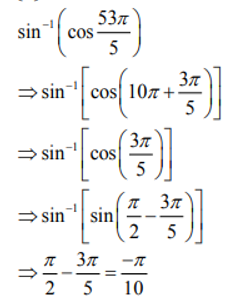5. If 3 tan–1 x + cot–1 x = π then x equal to

1. a. 0
2. b. 1
3. c. –1
4. d. ½

Solution:

3tan-1 x + cot-1x= π

⇒2tan-1x + (π/2) = π

⇒2tan-1x = π/2

⇒tan-1x = π/4

⇒tan-1 (π/4)= 1

6. The simplified form of

$$tan^{-1}\left [ \frac{x}{y} \right ]-tan^{-1}\left [ \frac{x-y}{x+y} \right ]$$
is equal to

1. a. 0
2. b. π/4
3. c. π/2
4. d. π

Solution:

$$\Rightarrow \tan ^{-1}\left(\frac{x}{y}\right)-\tan ^{-1}\left(\frac{x-y}{x+y}\right)^{-1}$$

$$\Rightarrow \tan ^{-1}\left(\frac{x}{y}\right)-\tan ^{-1}\left(\frac{1-\frac{y}{x}}{1+\frac{y}{x}}\right)$$

$$\Rightarrow \tan ^{-1}\left(\frac{x}{y}\right)-\left[\tan ^{-1} 1-\tan ^{-1} \frac{y}{x}\right]$$

$$\Rightarrow \tan ^{-1}\left(\frac{x}{y}\right)+\tan ^{-1}\left(\frac{y}{x}\right)-\tan ^{-1} 1$$

$$\Rightarrow \tan ^{-1}\left(\frac{x}{y}\right)+\cot ^{-1}\left(\frac{x}{y}\right)-\frac{\pi}{4}$$

$$\Rightarrow \frac{\pi}{2}-\frac{\pi}{4}=\frac{\pi}{4}$$

7. If x y z are all different and not equal to zero and

$$\begin{vmatrix} 1+x & 1 & 1\\ 1 & 1+y & 1\\ 1& 1 & 1+z \end{vmatrix}=0$$
then the value of x-1+ y-1+ z-1 is equal to

1. a. xyz
2. b. x-1y-1z-1
3. c. –x-y-z
4. d. -1

Solution:

$$\begin{vmatrix} 1+x & 1 & 1\\ 1 & 1+y & 1\\ 1& 1 & 1+z \end{vmatrix}=0$$

⇒ (1+x)[(1+y)(1+z)-1]-[1+z-1]+[1-1-y]=0

⇒ (1+x)[(1+y)(1+z)-(1+x)-z-y = 0

⇒ 1+x+y + z +xy+xz+yz+xyz=x+y+z+1

⇒ xy+yz+zx = -xyz

⇒(1/x) + (1/y) +(1/z) =-1

⇒ x-1+y-1+z-1 = -1

8. If A is any square matrix of order 3×3 then |3A| is equal to

1. a. 3|A|
2. b. 1/3| A |
3. c. 27|A|
4. d. 9|A|

Solution:

Use property |KA|= Kn|A| (here n = order of matrix)

⇒ |3A|= 33|A| = 27 |A|

9. If

$$y=e^{sin^{-1}(t^{2}-1)}$$
and
$$x=e^{sec^{-1}(\frac{1}{t^{2}-1})}$$
then dy/dx is equal to

1. a. x/y
2. b. –y/x
3. c. y/x
4. d. –x/y

Solution:

$$y=e^{sin^{-1}(t^{2}-1)}$$
;
$$x=e^{sec^{-1}(\frac{1}{t^{2}-1})}$$

$$y=e^{sin^{-1}(t^{2}-1)}$$
;
$$x=e^{cot^{-1}(t^{2}-1)}$$

$$xy=e^{sin^{-1}(t^{2}-1)}e^{cot^{-1}(t^{2}-1)}$$

$$xy=e^{sin^{-1}(t^{2}-1)+{cot^{-1}(t^{2}-1)}}$$

$$xy=e^\frac{\pi }{2}$$

On differentiating both side with respect to x

$$y+x\frac{dy}{dx}=0$$

$$\frac{dy}{dx}=\frac{-y}{x}$$

10. If

$$A=\frac{1}{\pi }\begin{bmatrix} sin^{-1}(\pi x) & tan^{-1}(\pi x) \\ sin^{-1}(\frac{x}{\pi })& cot^{-1}(\pi x) \end{bmatrix}$$
and
$$B=\frac{1}{\pi }\begin{bmatrix} -cos^{-1}(\pi x) & tan^{-1}(\pi x) \\ sin^{-1}(\frac{x}{\pi })& -tan^{-1}(\pi x) \end{bmatrix}$$
then A-B is equal to

1. a. I
2. b. 0
3. c. 2I
4. d. (1/2)I

Solution: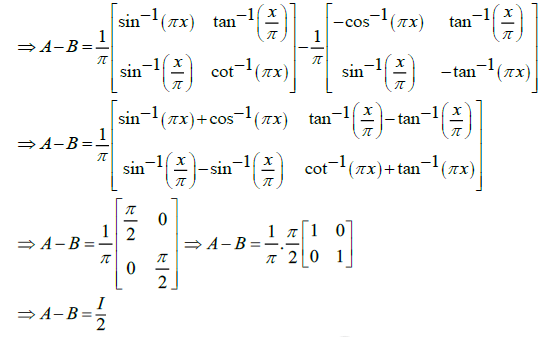11. If xy = e x-y then dy/dx is equal to

1. a.
$$\frac{logx}{log(x-y)}$$
2. b.
$$\frac{e^{x}}{x^{(x-y)}}$$
3. c.
$$\frac{log{x}}{(1+log x)^{2}}$$
4. d.
$$\frac{1}{y}-\frac{1}{x-y}$$

Solution:

xy =ex-y

on taking log both sides

$$ylog x=x-y\Rightarrow y=\frac{x}{1+logx}$$
--------(1)

On differentiating both side

$$\Rightarrow \frac{y}{x}+\log x \frac{d y}{d x}=1-\frac{d y}{d x}$$

$$\Rightarrow \frac{d y}{d x}(\log x+1)=1-\frac{y}{x}$$

Put value of y from eq. (1)

$$\Rightarrow \frac{d y}{d x}(1+\log x)=1-\frac{\frac{x}{(1+\log x)}}{x}$$

$$\Rightarrow \frac{d y}{d x}(1+\log x)=\frac{1+ \log \; x-1}{(1+\log x)}$$

$$\Rightarrow \frac{d y}{d x}=\frac{\log x}{(1+\log x)^2}$$

12.If A is a matrix of order m × n and B is a matrix such that AB' and B'A are both defined, the order of the matrix B is

1. a. m × m
2. b. n × n
3. c. n × m
4. d. m × n

Solution:

Given order of A is = m × n

Let the order of B is = v × u

If AB’ is defined = Amxn x B’uxv

This is possible when n = u

If B’A is defined = B’uxv x Amxn

This is possible when v = m

The order of B = vxu =m x n

13. The value of

$$\int \frac{e^{x}(1+x)dx}{cos^{2}(e^{x}.x)}$$
is equal to

1. a. –cot (e xx)+c
2. b. tan (ex. x)+c
3. c. tan(ex)+c
4. d. cot(ex)+c

Solution:

$$\int \frac{e^{x}(1+x)}{cos^{2}(e^{x}x)}dx$$

Since ex x =t

ex(1+x) dx =dt

$$\int \frac{dt}{cos^{2}t}$$

$$\int sec^{2}tdt=tant+x\Rightarrow tan(e^{x}x)+c$$

14. If x, y, z are not equal and ≠ 0, ≠ 1 the value of

$$\begin{vmatrix} logx & logy & log z\\ log 2x & log 2y & log 2z\\ log 3x & log 3y & log 3z \end{vmatrix}$$
is equal to

1. a. log(x y z)
2. b. log (6 x y z )
3. c. 0
4. d. log(x+ y+ z)

Solution:

$$\begin{vmatrix} logx & logy & log z\\ log 2x & log 2y & log 2z\\ log 3x & log 3y & log 3z \end{vmatrix}$$

R2 -> R2 - R1; R3 -> R3 - R1

$$\begin{vmatrix} logx & logy & log z\\ log 2 & log 2 & log 2\\ log 3 & log 3 & log 3 \end{vmatrix}$$

$$log 2 \, log 3\begin{vmatrix} logx & logy & log z\\ 1 & 1 & 1\\ 1 & 1 & 1 \end{vmatrix}$$

= 0 {because two row's are same}

15. The function f(x) = [x] where [x] the greatest integer function, is continuous at

1. a. 1.5
2. b. 4
3. c. 1
4. d. –2

Solution:

(f) = [x ]

As we know Greatest Integer Functions are discontinuous at all integers

$$LHL = \lim_{x\rightarrow 1.5^{-}}\left [ x \right ]=1$$

$$RHL = \lim_{x\rightarrow 1.5^{+}}\left [ x \right ]=1$$

F (1.5) = [1.5] =1

LHL=RHL=f (1.5)

Function f ( x ) is continuous at x = 1.5

16. The value of

$$\int \frac{e^{x}(x^{2}tan^{-1}x+tan^{-1}x+1)}{x^{2}+1}dx$$
is equal to

1. a. extan-1x+ c
2. b. tan-1(ex)+ c
3. c. tan-1(xe)+ c
4. d. etan-1x+ c

Solution:

$$\int \frac{e^{x}(x^{2}tan^{-1}x+tan^{-1}x+1)}{x^{2}+1}dx$$

$$\int {e^{x}(tan^{-1}x+\frac{1}{1+x^{2}})}dx$$

⇒ extan-1x+c

17. If

$$2\vec{a}.\vec{b}=\left | \vec{a} \right |.\left | \vec{b} \right |$$
then the angle between
$$\vec{a}$$
and
$$\vec{b}$$
is

1. a. 30°
2. b. 0°
3. c. 90°
4. d. 60°

Solution:

$$2\vec{a}.\vec{b}=\left | \vec{a} \right |.\left | \vec{b} \right |$$

$$\Rightarrow 2\left | \vec{a} \right |.\left | \vec{b} \right |cos\theta =\left | \vec{a} \right |.\left | \vec{b} \right |$$

$$\Rightarrow cos\theta =\frac{1}{2}\Rightarrow \theta =\frac{\pi }{3}$$

18. If xmyn =(x+y)(m+n) then dy/dx is equal to

1. a. (x + y)/xy
2. b. xy
3. c. 0
4. d. y/x

Solution: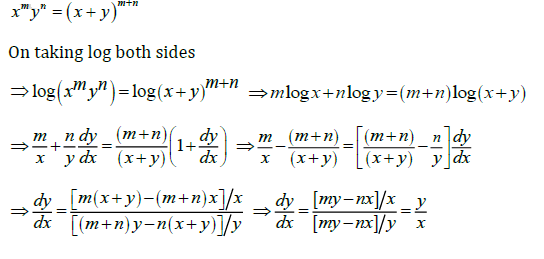19. The general solution of cot θ +tan θ =2 is

1. a.
$$\theta =\frac{n\pi }{2}+\left ( -1 \right )^{n}\frac{\pi }{8}$$
2. b.
$$\theta =\frac{n\pi }{2}+\left ( -1 \right )^{n}\frac{\pi }{4}$$
3. c.
$$\theta =\frac{n\pi }{2}+\left ( -1 \right )^{n}\frac{\pi }{6}$$
4. d.
$$\theta =n\pi +\left ( -1 \right )^{n}\frac{\pi }{8}$$

Solution: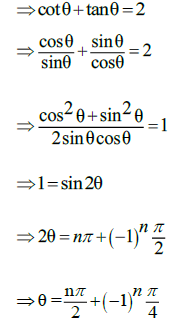20. The value of

$$\int_{-\pi /4}^{\pi /4}sin^{103}x.cos^{101}xdx$$
is

1. a.
$$\left [ \frac{\pi }{4} \right ]^{103}$$
2. b.
$$\left [ \frac{\pi }{4} \right ]^{101}$$
3. c. 2
4. d. 0

Solution:

$$I=\int_{-\pi /4}^{\pi /4}sin^{103}x.cos^{101}xdx$$
---------(1)

Put x = -x

$$I=-\int_{-\pi /4}^{\pi /4}sin^{103}x.cos^{101}xdx$$
------(2)

We can say that the given function is an add function

2I = 0

I = 0

21. The length of latus rectum of the parabola 4y2+3x + 3y + 1 = 0 is

1. a. 4/3
2. b. 7
3. c. 12
4. d. 3/4

Solution:

4y2+3y=-3x-1

$$4\left [ y^{2}+\frac{3}{4}y \right ]=-3x-1$$

$$4\left [ y^{2}+\frac{3}{4}y +\frac{9}{64}-\frac{9}{64}\right ]=-3x-1$$

$$4\left [ \left ( y+\frac{3}{8} \right )^{2} -\frac{9}{64}\right ]=-3x-1$$

$$\left [ \left ( y+\frac{3}{8} \right ) \right ]^{2}=\frac{-3x-1}{4}+\frac{9}{64}$$

$$\left [ \left ( y+\frac{3}{8} \right ) \right ]^{2}=\frac{-3x}{4}-\frac{7}{64}=\frac{-3}{4}\left [ x+\frac{7}{48} \right ]$$

$$\Rightarrow LLR=4a=\left | 4\times \left ( \frac{-3}{4\times 4} \right ) \right |=\frac{-3}{4}=\frac{3}{4}$$

22. The value of

$$\int \frac{e^{6logx}-e^{5logx}}{e^{4logx}-e^{3logx}}$$
dx is equal to

1. a. 0
2. b.
$$\frac{x^{3}}{3}+c$$
3. c.
$$\frac{3}{x^{3}}+c$$
4. d.
$$\frac{1}{x}+c$$

Solution:

$$\int \frac{e^{6logx}-e^{5logx}}{e^{4logx}-e^{3logx}}$$
dx

$$\int \frac{{x^{6}}-x^{5}}{x^{4}-x^{3}}dx$$

$$\Rightarrow \int \frac{x^{5}(x-1)}{x^{3}(x-1)}dx\Rightarrow \int x^{2}dx\Rightarrow \frac{x^{3}}{3}+C$$

23. The differential coefficient of log10 x with respect to log x10 is

1. a. 1
2. b. –( log10 x )2
3. c. ( log x 10 )2
4. d. x2/100

Solution:

let u = log10 x

$$v=log_{x}10=\frac{1}{log_{10}x}=\frac{1}{u}$$
-------------------(1)

⇒ vu =1

On differentiating both side with respect to v.

$$\Rightarrow \frac{du}{dv}=\frac{-u}{v}$$
(from eq.(1) v =1/u)

$$\Rightarrow \frac{du}{dv}=-u^{2}=-(log_{10}x)^{2}$$

24. The slope of the tangent to the curve x=t2+ 3t – 8, y =2t2–2t–5 at the point (2,–1) is

1. a. 22/7
2. b. 6/7
3. c. 7/6
4. d. –6/7

Solution:

X=t2+3t-8

Y = 2t2-2t-5

Substitute x = 2 in given eq.

2 =t2+3t -8

t = -5,2

Now substitute y = -1

-1=2t2-2t-5

t = 2,-1

Common value of t is 2.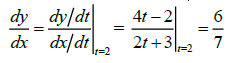25. The real part of (1–cosθ +i sin θ)–1 is

1. a. 1/2
2. b. 1/(1+cosθ)
3. c. tanθ/2
4. d. cot θ/2

Solution:

$$\Rightarrow(1-\cos \theta+i \sin \theta)^{-1}$$

$$\Rightarrow \frac{1}{(1-\cos \theta+i \sin \theta)} \times \frac{(1-\cos \theta-i \sin \theta)}{(1-\cos \theta-i \sin \theta)} \Rightarrow \frac{(1-\cos \theta)-i \sin \theta}{(1-\cos \theta)^{2}+\sin ^{2} \theta}$$

$$\Rightarrow \frac{(1-\cos \theta)-i \sin \theta}{4 \sin ^{4} \frac{\theta}{2}+4 \sin ^{2} \frac{\theta}{2} \cos ^{2} \frac{\theta}{2}} \Rightarrow \frac{2 \sin ^{2} \frac{\theta}{2}-i\left[2 \sin \frac{\theta}{2} \cos \frac{\theta}{2}\right]}{4 \sin ^{2} \frac{\theta}{2}\left[\sin ^{2} \frac{\theta}{2}+\cos ^{2} \frac{\theta}{2}\right]}$$

$$\Rightarrow \frac{\sin \frac{\theta}{2}-i \cos \frac{\theta}{2}}{2 \sin \frac{\theta}{2}} \Rightarrow {Re}\left(\frac{1}{2}-\frac{1}{2} i \cot \frac{\theta}{2}\right) \Rightarrow \frac{1}{2}$$

26.

$$\int_{0}^{\pi / 2} \frac{\sin ^{1000} x d x}{\sin ^{1000} x+\cos ^{1000} x}$$
is equal to

1. a. 1000
2. b. 1
3. c. π/2
4. d. π/4

Solution:

$$I=\int_{0}^{\pi / 2} \frac{\sin ^{1000} x d x}{\sin ^{1000} x+\cos ^{1000} x}$$
------------(1)

Apply king property

$$I=\int_{0}^{\pi / 2} \frac{\cos^{1000} x d x}{\cos ^{1000} x+\sin ^{1000} x}$$
--------------(2)

On adding eq. (1) and eq. (2)

$$2I=\int_{0}^{\pi / 2} 1.dx$$

$$I=\frac{\pi }{4}$$

27. If 1+sinθ+sin2θ+------upto ∞=2√3+4 the θ =

1. a. π/6
2. b. π/4
3. c. π/3
4. d. 3π/4

Solution: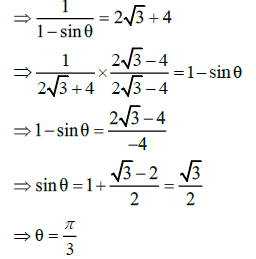28.

$$\lim _{x \rightarrow 0} \frac{x e^{x}-\sin x}{x}$$
is equal to

1. a. 3
2. b. 1
3. c. 0
4. d. 2

Solution:

$$\lim _{x \rightarrow 0} \frac{x e^{x}-\sin x}{x}\left ( \frac{0}{0} \right )$$

L-Hospital Rule

$$\lim _{x \rightarrow 0} \frac{x e^{x}+e^{x}-\cos x}{x}=0+1+1=0$$

29. If tan-1(x2+y2) = α then dy/dx is equal to

1. a. –x/y
2. b. xy
3. c. x/y
4. d. –xy

Solution:

tan-1(x2+y2)=α

(x2+y2)=tan α

2x+2y(dy/dx) =0

(dy/dx) = (-x/y)

30. The simplified form of in+In+1+In+2+In+3 is

1. a. 0
2. b. 1
3. c. –1
4. d. i

Solution:

in+In+1+In+2+In+3

= (i)n+i(i)n –(i)n-i(i)n

= 0

31. The two curves x3 – 3xy2 + 2 = 0 and 3x2y – y3 = 2

1. a. Touch each other
2. b. Cut each other at right angle
3. c. Cut at an angle π/3
4. d. Cut at an angle π/4

Solution:32. The equation of the normal to the curve y(1 + x2) = 2 – x where the tangent crosses x-axis is

1. a. 5x – y – 10 = 0
2. b. x – 5y – 10 = 0
3. c. 5x + y + 10 = 0
4. d. x + 5y + 10 = 0

Solution:

Tangent crosses x-axis.

Put y = 0 ⇒ x = 2

$$\left.\frac{d y}{d x}\right|_{(2,0)}=\frac{-1-2 x y}{\left(1+x^{2}\right)} \\$$

$$\left.\frac{d y}{d x}\right|_{(2,0)}=\frac{-1}{5}=m_{T}$$

mN =5

Eq. of normal at (2, 0) is y – 0 = 5( x – 2)

Or 5 x –y =10

33. The maximum value of (1/x)x is

1. a. e
2. b. ee
3. c. e 1/e
4. d. (1/e)e

Solution:

$$\Rightarrow y=\frac{1}{x^{x}}$$

⇒ log y = -x log x

$$\frac{1}{y}\frac{dy}{dx}=\frac{-x}{x}+(-logx)$$

$$\frac{dy}{dx}=-x^{-x}(1+logx)=0$$

⇒ 1+log x =0

⇒ x = (1/e)(point of maxima)

max (y) = e 1/e

34. The solution for the differential equation (dy/y)+ (dx/y) = 0 is.

1. a. (1/y)+(1/x) =c
2. b. log x.log y =c
3. c. xy = c
4. d. x+y = c

Solution:

$$\frac{dy}{y}+\frac{dx}{x}=0$$

On integrating both sides

⇒log y +log x + log c

⇒xy =c

35. The order and degree of the differential equation

$$1+(\frac{dy}{dx})^{2}+sin(\frac{dy}{dx})^{\frac{3}{4}}=(\frac{d^{2}y}{dx^{2}})$$

1. a. Order =2,Degree = 3
2. b. Order =2,Degree = 4
3. c. Order =2,Degree =3/4

Solution:

$$1+(\frac{dy}{dx})^{2}+sin(\frac{dy}{dx})=(\frac{d^{2}y}{dx^{2}})^{\frac{4}{3}}$$

Order = 2

Degree = not defined (because of sin dy/dx)

36. If

$$\vec{a}$$
and
$$\vec{b}$$
are unit vectors then what is the angle between
$$\vec{a}$$
and
$$\vec{b}$$
for
$$\sqrt{3}\vec{a}-\vec{b}$$
to be unit vector?

1. a. 30°
2. b. 45°
3. c. 60°
4. d. 90°

Solution:

$$\left |\sqrt{3}\vec{a}-\vec{b} \right |=1$$

On squaring both sides

$$\Rightarrow 3\left | \vec{a} \right |^{2}+\left | \vec{b} \right |^{2}-2\sqrt{3}\left | \vec{a} \right |\left | \vec{b} \right |cos\theta =1$$

$$\Rightarrow cos\alpha =\frac{3}{2\sqrt{3}}=\frac{\sqrt{3}}{2}$$

$$\Rightarrow \theta =\frac{\pi }{6}$$

37. The sum of 1st n terms of the series

$$\frac{1^{2}}{1}+\frac{1^{2}+2^{2}}{1+2}+\frac{1^{2}+2^{2}+3^{2}}{1+2+3}+.....$$

1. a.
$$\frac{1^{2}}{1}+\frac{1^{2}+2^{2}}{1+2}+\frac{1^{2}+2^{2}+3^{2}}{1+2+3}+.....$$
2. b.
$$\frac{n(n+2)}{3}$$
3. c.
$$\frac{n(n-2)}{3}$$
4. d.
$$\frac{n(n-2)}{6}$$

Solution: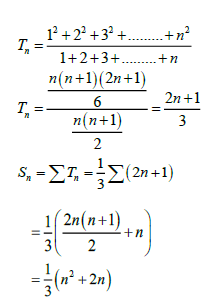38. The 11th term in the expansion of

$$\left [ x+\frac{1}{\sqrt{x}} \right ]^{14}$$
is

1. a. 999/x
2. b. 1001/x
3. c. i
4. d. 1001

Solution:

$$T_{11}=^{14}C_{10}(x)^{14-10}\left [ \frac{1}{\sqrt{x}} \right ]^{10}$$

$$T_{11}=^{14}C_{10}(x)^4\frac{1}{x^{5}}$$

= 1001/x

39. Suppose

$$\vec{a}+\vec{b}+\vec{c}=0,\left | \vec{a} \right |=3,\left | \vec{b} \right |=5,\left | \vec{c} \right |=7$$
, then the angle between
$$\vec{a}$$
&
$$\vec{b}$$
is

1. a. π
2. b. π/2
3. c. π/3
4. d. π/4

Solution:

$$\Rightarrow \vec{a}+\vec{b}+\vec{c}=0$$

$$\Rightarrow \left | \vec{a}+\vec{b}\right |^{2}=\left | -\vec{c} \right |^{2}$$

$$\Rightarrow \left | \vec{a}\right |^{2}+\left | \vec{b}\right |^{2}+2\left | \vec{a} \right |\left | \vec{b} \right |cos\theta =\left | \vec{c} \right |^{2}$$

⇒ 9+25 +2x15 cosθ=49

⇒ 30 cosθ=15

⇒ cos θ=1/2

⇒ θ= π/3

40. If a = 3, b = 4, c = 5 each one of

$$\vec{a}$$
,
$$\vec{b}$$
and
$$\vec{c}$$
is perpendicular to the sum of the remaining then
$$\left | \vec{a} +\vec{b}+\vec{c}\right |$$
is equal to

1. a.
$$\frac{5}{\sqrt{2}}$$
2. b.
$$\frac{2}{\sqrt{5}}$$
3. c.
$$5\sqrt{2}$$
4. d.
$$\sqrt{5}$$

Solution: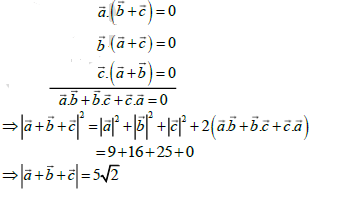41. If the straight lines 2x + 3y – 3 = 0 and x + ky + 7 = 0 are perpendicular, then the value of k is

1. a. 2/3
2. b. 3/2
3. c. –2/3
4. d. –3/2

Solution:

$$m_{1}=\frac{-2}{3}$$

$$m_{2}=\frac{-1}{k}$$

⇒ m1m2 =-1

$$\Rightarrow \frac{2}{3K}=-1\Rightarrow K=\frac{-2}{3}$$

42. The rate of change of area of a circle with respect to its radius at r =2 cms is

1. a. 4
2. b. 2 π
3. c. 2
4. d. 4 π

Solution:

A= πr2

$$\left.\Rightarrow \frac{d A}{d r}\right|_{r=2}=2 \pi r=2 \pi \times 2=4 \pi$$

43. The value of tan (π/8) is equal to

1. a. ½
2. b.
$$\sqrt{2}+1$$
3. c.
$$\frac{1}{\sqrt{2}+1}$$
4. d.
$$1-\sqrt{2}$$

Solution:

$$\Rightarrow \tan \frac{\pi}{8}$$

$$\Rightarrow \frac{\sin 2(\pi / 8)}{1+\cos 2(\pi / 8)}$$

$$\Rightarrow \frac{\sin \pi / 4}{1+\cos \pi / 4} \Rightarrow \frac{1}{1+\sqrt{2}}$$

44. Area lying between the curves y2 =2x and y = x is

1. a. (2/3)sq.units
2. b. (1/3)sq.units
3. c. (1/4)sq. units
4. d. (3/4)sq. units

Solution: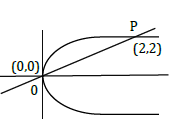x2= 2x

x =0 , x =2

y =0,y =2

$$\Rightarrow \int_{0}^{2}(\sqrt{2} \sqrt{x}-x) d x$$

$$\Rightarrow \sqrt{2}\left[\frac{2 x^{3 / 2}}{3}\right]_{0}^{2}-\left[\frac{x^{2}}{2}\right]_{0}^{2}$$

Or 8/3 - 2 = 2/3 sq. units

45. If If P (Aᴒ B) =7/10 and P (B) = 17/20 , where P stands for probability then P (A|B) is equal to

1. a. 7/8
2. b. 17/20
3. c. 14/17
4. d. 1/8

Solution:

$$p(\frac{A}{B})=\frac{P(A\cap B)}{P(B)}=\frac{7/10}{17/20}$$

=14/17

46. The coefficient of variation of two distributions are 60 and 70.The standard deviation are 21 and 16 respectively, then their mean is

1. a. 35
2. b. 23
3. c. 28.25
4. d. 22.85

Solution: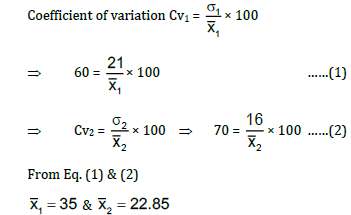47. Two cards are drawn at random from a pack of 52 cards. The probability of these two being "Aces" is

1. a. 1/26
2. b. 1/221
3. c. 1/2
4. d. 1/13

Solution:

Probability of two cards being aces is =(4C2/52C2) =1/221

48. If

$$sin^{-1}x+sin^{-1}y=\frac{\pi }{2}$$
then x2 is equal to

1. a. 1-y2
2. b. y2
3. c. 0
4. d.
$$\sqrt{1-y}$$

Solution:

$$sin^{-1}x+sin^{-1}y=\frac{\pi }{2}$$

$$\Rightarrow sin^{-1}x=\frac{\pi }{2}-sin^{-1}y$$

$$\Rightarrow sin^{-1}x=cos^{-1}y$$

$$\Rightarrow sin^{-1}x=sin^{-1}\sqrt{1-y^{2}}$$

⇒ x2 = 1-y2

49. The value of

$$\int_{2}^{8}\frac{\sqrt{10-x}}{\sqrt{x}+\sqrt{10-x}}dx$$
is

1. a. 10
2. b. 0
3. c. 8
4. d. 3

Solution:

$$I=\int_{2}^{8}\frac{\sqrt{10-x}}{\sqrt{x}+\sqrt{10-x}}dx$$
---------(1)

$$I=\int_{2}^{8}\frac{\sqrt{x}}{\sqrt{x}+\sqrt{10-x}}dx$$
------------(2)

On adding eq. (1) and eq. (2)

$$2I=\int_{2}^{8}Idx=6\Rightarrow I=3$$

50. The contra positive of the converse of the statement "If x is a prime number then x is odd" is

1. a. If x is not a prime number then x is odd.
2. b. If x is not an odd number then x is not a prime number.
3. c. If x is a prime number then it is not odd.
4. d. If x is not a prime number then x is not an odd.

Solution:

The converse of the given statement p -> q is q -> p

The contra positive of the given statement is q -> p is p -> q

Hence, the contra positive of the converse of the given statement is “ If x is not a prime number, then x is not odd”

51. Two dice are thrown simultaneously, the probability of obtaining a total score of 5 is

1. a. 1/18
2. b. 1/12
3. c. 1/9
4. d. 1/6

Solution:

Total ways of obtaining score of 5 is {(1, 4), (2, 3), (3, 2), (4, 1)}

Required probability is = 4/62 =4/36 =1/9

52. If A =

$$\begin{bmatrix} cos2\theta & -sin2\theta \\ sin2\theta & cos2\theta \end{bmatrix}$$
and A+AT =I, Where I is the unit matrix of 2×2 & AT is the transpose of A, then the value of θ is equal to.

1. a. π/6
2. b. π/3
3. c. π
4. d. 3π/2

Solution:

A+AT =I

$$\Rightarrow \begin{bmatrix} cos2\theta & -sin2\theta \\ sin2\theta & cos2\theta \end{bmatrix}+\begin{bmatrix} cos2\theta & sin2\theta \\ -sin2\theta & cos2\theta \end{bmatrix}=\begin{bmatrix} 1 & 0\\ 0& 1 \end{bmatrix}$$

$$\begin{bmatrix} 2cos2\theta & 0 \\ o & 2cos2\theta \end{bmatrix}=\begin{bmatrix} 1 & 0\\ 0& 1 \end{bmatrix}$$

2cos2θ = 1

cos2θ = 1/2

2θ = π/3

θ = π/6

53. If

$$A=\begin{bmatrix} 3 & 1 \\ -1& 2 \end{bmatrix}$$
then A2-5A is equal to

1. a. I
2. b. -I
3. c. 7I
4. d. -7I

Solution:

$$\left | A-\lambda I \right |=0$$

$$\begin{bmatrix} 3-\lambda & 1 \\ -1& 2-\lambda \end{bmatrix}=0$$

⇒ (λ-2)( (λ-3)+1 =0

⇒ λ2 -5 λ+6+1=0

⇒ λ = A

⇒A2- 5A+7I=0

⇒ A2- 5A = -7I

54. The value of x if

$$x\left ( \hat{i}+\hat{j}+\hat{k}\right )$$
is a unit vector is

1. a.
$$\pm \frac{1}{\sqrt{3}}$$
2. b.
$$\pm \sqrt{3}$$
3. c.
$$\pm 3$$
4. d.
$$\pm \frac{1}{3}$$

Solution:

$$x\left ( \hat{i}+\hat{j}+\hat{k}\right )$$
=1

$$\Rightarrow \sqrt{x^{2}+x^{2}+x^{2}}=1$$

$$\Rightarrow \sqrt{3x^{2}}=1$$

$$x=\pm \frac{1}{\sqrt{3}}$$

55. If x = 2 + 3 cos θ and y = 1 – 3 sin θ represent a circle then the Centre and radius is

1. a. (2,1), 9
2. b. (2,1), 3
3. c. (1, 2), (1/3)
4. d. (-2, -1), 3

Solution:

x = 2 + 3cosθ

$$\Rightarrow \left ( \frac{x-2}{3} \right )=cos\theta$$
------------(i)

y = 1- 3cosθ

$$\Rightarrow \left ( \frac{y-2}{-3} \right )=sin\theta$$
----------(ii)

Equation (1)2 + Equation (2)2

⇒ sin2θ+cos2 θ =

$$\left ( \frac{x-2}{3} \right )^{2} + \left ( \frac{y-2}{-3} \right )^{2}$$

⇒ (x-2)2+(y-1)2 =9

Centre = (2, 1) ; radius = 3

56. The vector equation of the plane which is at a distance of

$$\frac{3}{\sqrt{14}}$$
from the origin and the normal from the origin is
$$2\hat{i}-3\hat{j}+\hat{k}$$
is

1. a.
$$\vec{r}.(2\hat{i}-3\hat{j}+\hat{k})=3$$
2. b.
$$\vec{r}.(\hat{i}+\hat{j}+\hat{k})=9$$
3. c.
$$\vec{r}.(\hat{i}+2\hat{j})=3$$
4. d.
$$\vec{r}.(2\hat{i}+\hat{k})=3$$

Solution:

Vector equation of a plane is

$$\vec{r}.\hat{n}=d$$

$$\Rightarrow \vec{r}(\frac{(2\hat{i}-3\hat{j}+\hat{k})}{\sqrt{14}}=\frac{3}{\sqrt{14}}$$

$$\Rightarrow \vec{r}(2\hat{i}-3\hat{j}+\hat{k})=3$$

57. Find the co-ordinates of the foot of the perpendicular drawn from the origin to the plane 5y + 8 =0:

1. a.
$$\left [ 0,-\frac{18}{5},2 \right ]$$
2. b.
$$\left [ 0,\frac{8}{5},0 \right ]$$
3. c.
$$\left [ \frac{8}{25},0,0 \right ]$$
4. d.
$$\left [ 0,-\frac{8}{5},0 \right ]$$

Solution:

Let foot of perpendicular drawn from origin is (x1, y1, z1)

$$\Rightarrow \frac{x_{1}-0}{0}=\frac{y_{1}-0}{5}=\frac{z_{1}-0}{0}=\frac{-(0+0+0+8))}{0^{2}+5^{2}+0^{2}}$$

$$\Rightarrow \frac{x_{1}}{0}=\frac{y_{1}}{5}=\frac{z_{1}}{0}=\frac{-8}{25}$$

x1=0, y1= (-8/5), z1=0

Co-ordinate is = (0, -8/5 ,0)

58. If cosα, cosβ,cosγ are the direction cosines of a vector a then cos2α + cos2β+ cos2γ is equal to

1. a. 2
2. b. 3
3. c. –1
4. d. 0

Solution:

As we know that

cos2α + cos2β+ cos2γ =1

$$\Rightarrow \frac{1+cos2\alpha }{2}+\frac{1+cos2\beta }{2}+\frac{1+cos2\gamma }{2}=1$$

cos2α + cos2β+ cos2γ = -1

59. The value of the sin1° + sin2° +……. + sin 359° is equal to

1. a. 0
2. b. 1
3. c. –1
4. d. 180

Solution:

sin1° + sin2° +……. + sin 359°

(sin1° + sin 359°) + (sin2°+ sin 358°)+----------+sin 1800

(sin1° - sin1°)+ (sin2° - sin2°) + ---------+ sin 1800

60. Integrating factor of

$$x\frac{dy}{dx}-y=x^{4}-3x$$
is

1. a. x
2. b. log x
3. c. 1/x
4. d. –x

Solution:

$$x\frac{dy}{dx}-y=x^{4}-3x$$

$$\frac{dy}{dx}-\frac{y}{x}=x^{3}-3$$

L.F =

$$e^{-\int \frac{1}{x}dx}=e^{-Inx}=\frac{1}{x}$$

### KCET 2016 Mathematics Question Paper with Solutions